# AP SSC 10th Class Maths Solutions Chapter 4 Pair of Linear Equations in Two Variables Ex 4.3

AP State Board Syllabus AP SSC 10th Class Maths Textbook Solutions Chapter 4 Pair of Linear Equations in Two Variables Ex 4.3 Textbook Questions and Answers.

## AP State Syllabus SSC 10th Class Maths Solutions 4th Lesson Pair of Linear Equations in Two Variables Exercise 4.3

### 10th Class Maths 4th Lesson Pair of Linear Equations in Two Variables Ex 4.3 Textbook Questions and Answers

Question 1.
Solve each of the following pairs of equations by reducing them to a pair of linear equations.
i) $$\frac{5}{x-1}$$ + $$\frac{1}{y-2}$$ = 2
$$\frac{6}{x-1}$$ + $$\frac{3}{y-2}$$ = 1
Given
$$\frac{5}{x-1}$$ + $$\frac{1}{y-2}$$ = 2
$$\frac{6}{x-1}$$ + $$\frac{3}{y-2}$$ = 1
Put $$\frac{1}{x-1}$$ = a and $$\frac{1}{y-2}$$ = b,
then the given equations reduce to
5a + b = 2 ……… (1)
6a – 3b = 1 ………. (2)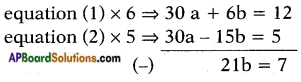⇒ b = $$\frac{7}{21}$$ = $$\frac{1}{3}$$
Substituting b = $$\frac{1}{3}$$ in equation (1) we get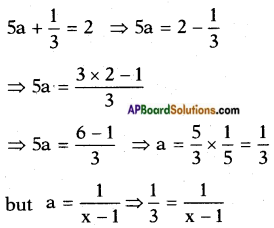⇒ (x – 1) . 1 = 3 × 1
⇒ x – 1 = 3
⇒ x = 3 + 1 = 4
b = $$\frac{1}{y-2}$$ ⇒ $$\frac{1}{3}$$ = $$\frac{1}{y-2}$$
⇒ (y – 2) . 1 = 3 × 1
⇒ y – 2 = 3
⇒ y = 3 + 2 = 5
∴ Solution (x, y) = (4, 5)ii) $$\frac{x+y}{xy}$$ = 2;
$$\frac{x-y}{xy}$$ = 6
Given
$$\frac{x+y}{xy}$$ = 2
⇒ $$\frac{x}{xy}$$ + $$\frac{y}{xy}$$ = 2
⇒ $$\frac{1}{y}$$ + $$\frac{1}{x}$$ = 2
$$\frac{x-y}{xy}$$ = 6
⇒ $$\frac{x}{xy}$$ – $$\frac{y}{xy}$$ = 6
⇒ $$\frac{1}{y}$$ – $$\frac{1}{x}$$ = 6
Take $$\frac{1}{x}$$ = a and $$\frac{1}{y}$$ = b,
then the given equations reduces to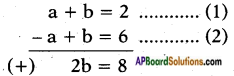⇒ b = $$\frac{8}{2}$$ = 4
Substituting b = 4 in equation (1) we get
a + 4 = 2 ⇒ a = 2 – 4 = -2
but a = $$\frac{1}{x}$$ = -2 ⇒ x = $$\frac{-1}{2}$$
b = $$\frac{1}{y}$$ = 4 ⇒ y = $$\frac{1}{4}$$
∴ Solution (x, y) = $$\left(\frac{-1}{2}, \frac{1}{4}\right)$$

iii) $$\frac{2}{\sqrt{x}}$$ + $$\frac{3}{\sqrt{y}}$$ = 2;
$$\frac{4}{\sqrt{x}}$$ – $$\frac{9}{\sqrt{y}}$$ = -1
Given
$$\frac{2}{\sqrt{x}}$$ + $$\frac{3}{\sqrt{y}}$$ = 2 and $$\frac{4}{\sqrt{x}}$$ – $$\frac{9}{\sqrt{y}}$$ = -1
Take $$\frac{1}{\sqrt{x}}$$ = a and $$\frac{1}{\sqrt{y}}$$ = b,
then the given equations reduces to
2a + 3b = 2 …….. (1)
4a – 9b = – 1 …….. (2)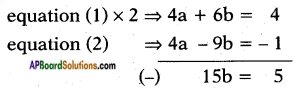⇒ b = $$\frac{5}{15}$$ = $$\frac{1}{3}$$
Substituting b = $$\frac{1}{3}$$ in equation (1) we get
2a + 3$$\left(\frac{1}{3}\right)$$ = 2
⇒ 2a + 1 = 2 ⇒ 2a = 2 – 1 ⇒ a = $$\frac{1}{2}$$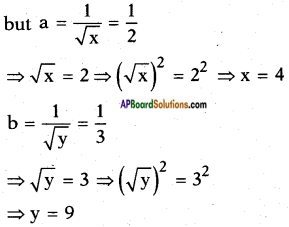∴ Solution (x, y) = (4, 9)iv) 6x + 3y = 6xy
2x + 4y = 5xy
Given
6x + 3y = 6xy
⇒ $$\frac{6x+3y}{xy}$$ = 6
⇒ $$\frac{6x}{xy}$$ + $$\frac{3y}{xy}$$ = 6
⇒ $$\frac{6}{y}$$ + $$\frac{3}{x}$$ = 6
2x + 4y = 5xy
⇒ $$\frac{2x+4y}{xy}$$ = 5
⇒ $$\frac{2x}{xy}$$ + $$\frac{4y}{xy}$$ = 6
⇒ $$\frac{2}{y}$$ + $$\frac{4}{x}$$ = 6
Take $$\frac{1}{x}$$ = a and $$\frac{1}{y}$$ = b,
then the given equations reduces to
3a + 6b = 6 ……. (1)
4a + 2b = 5 ……. (2)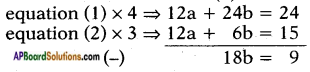⇒ b = $$\frac{9}{18}$$ = $$\frac{1}{2}$$
Substituting b = $$\frac{1}{2}$$ in equation (1) we get
3a +6$$\left(\frac{1}{2}\right)$$ = 6
⇒ 3a = 6 – 3
⇒ a = $$\frac{3}{3}$$ = 1
but a = $$\frac{1}{x}$$ = 1 ⇒ x = 1
b = $$\frac{1}{y}$$ = $$\frac{1}{2}$$ ⇒ y = 2
∴ Solution (x, y) = (1, 2)

v) $$\frac{5}{x+y}$$ – $$\frac{2}{x-y}$$ = -1
$$\frac{15}{x+y}$$ + $$\frac{7}{x-y}$$ = 10
where x ≠ 0, y ≠ 0
Given
$$\frac{5}{x+y}$$ – $$\frac{2}{x-y}$$ = -1 and
$$\frac{15}{x+y}$$ + $$\frac{7}{x-y}$$ = 10
Take $$\frac{1}{x+y}$$ = a and $$\frac{1}{x-y}$$ = b, then
the given equations reduce to
5a – 2b = – 1 ……… (1)
15a + 7b = 10 ……… (2)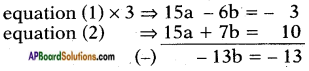⇒ b = $$\frac{-13}{-13}$$ = 1
Substituting b = 1 in equation (1) we get
5a – 2(1) = -1
⇒ 5a = -1 + 2
⇒ 5a = 1
⇒ a = $$\frac{1}{5}$$
but a = $$\frac{1}{x+y}$$ = $$\frac{1}{5}$$ ⇒ x + y = 5
b = $$\frac{1}{x-y}$$ = 1 ⇒ x – y = 1
⇒ x = $$\frac{6}{2}$$ = 3
Solving the above equations
Substituting x = 3 in x + y = 5 we get
3 + y = 5 ⇒ y = 5 – 3 = 2
∴ Solution (x, y) = (3, 2)vi) $$\frac{2}{x}$$ + $$\frac{3}{y}$$ = 13
$$\frac{5}{x}$$ – $$\frac{4}{y}$$ = -2
where x ≠ 0, y ≠ 0
Given
$$\frac{2}{x}$$ + $$\frac{3}{y}$$ = 13 and
$$\frac{5}{x}$$ – $$\frac{4}{y}$$ = -2
Take $$\frac{1}{x}$$ = a and $$\frac{1}{y}$$ = b, then
the given equations reduce to
2a + 3b = 13 ……… (1)
5a – 4b = -2 ……… (2)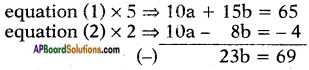⇒ b = $$\frac{69}{23}$$ = 3
Substituting b = 3 in equation (1) we get
2a + 3 (3) = 13
⇒ 2a = 13 – 9
⇒ a = $$\frac{4}{2}$$ = 2
but a = $$\frac{1}{x}$$ = 2 ⇒ x = $$\frac{1}{2}$$
b = $$\frac{1}{y}$$ = 3 ⇒ y = $$\frac{1}{3}$$
∴ Solution (x, y) = ($$\frac{1}{2}$$, $$\frac{1}{3}$$)

vii) $$\frac{10}{x+y}$$ + $$\frac{2}{x-y}$$ = 4
$$\frac{15}{x+y}$$ – $$\frac{5}{x-y}$$ = -2
Given
$$\frac{10}{x+y}$$ + $$\frac{2}{x-y}$$ = 4 and
$$\frac{15}{x+y}$$ – $$\frac{5}{x-y}$$ = -2
Take $$\frac{1}{x+y}$$ = a and $$\frac{1}{x-y}$$ = b, then
the given equations reduce to
10a + 2b = 4 ……… (1)
15a – 5b = – 2 ……… (2)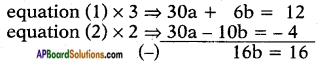⇒ b = $$\frac{16}{16}$$ = 1
Substituting b = 1 in equation (1) we get
10a + 2(1) = 4
⇒ 10a = 4 – 2
⇒ a = $$\frac{2}{10}$$ = $$\frac{1}{5}$$
but a = $$\frac{1}{x+y}$$ = $$\frac{1}{5}$$ ⇒ x + y = 5 ……. (3)
b = $$\frac{1}{x-y}$$ = 1 ⇒ x – y = 1 …….. (4)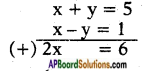⇒ x = $$\frac{6}{2}$$ = 3
Substituting x = 3 in x + y = 5 we get
3 + y = 5 ⇒ y = 5 – 3 = 2
∴ Solution (x, y) = (3, 2)viii) $$\frac{1}{3x+y}$$ + $$\frac{1}{3x-y}$$ = $$\frac{3}{4}$$
$$\frac{1}{2(3x+y)}$$ – $$\frac{1}{2(3x-y)}$$ = $$\frac{-1}{8}$$
Given
$$\frac{1}{3x+y}$$ + $$\frac{1}{3x-y}$$ = $$\frac{3}{4}$$ and
$$\frac{1}{2(3x+y)}$$ – $$\frac{1}{2(3x-y)}$$ = $$\frac{-1}{8}$$
Take $$\frac{1}{3x+y}$$ = a and $$\frac{1}{3x-y}$$ = b, then
the given equations reduce to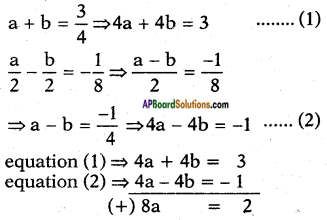⇒ a = $$\frac{2}{8}$$ = $$\frac{1}{4}$$
Substituting a = $$\frac{1}{4}$$ in equation (1) we get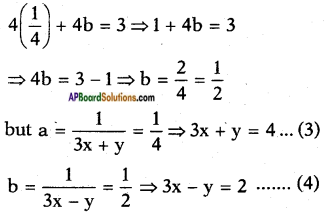Solving (3) and (4)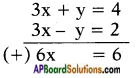⇒ x = $$\frac{6}{6}$$ = 1
Substituting x = 1 in 3x + y = 4
⇒ 3(1) + y = 4
⇒ y = 4 – 3 = 1
∴ The solution (x, y) = (1, 1)

Question 2.
Formulate the following problems as a pair of equations and then find their solutions.
i) A boat goes 30 km upstream and 44 km downstream in 10 hours. In 13 hours it can go 40 km upstream and 55 km downstream. Determine the speed of the stream and that of the boat in still water.
Let the speed of the boat in still water = x kmph
and the speed of the stream = y kmph
then speed in downstream = x + y
Speed in upstream = x – y
and time = $$\frac{\text { distance }}{\text { speed }}$$
By problem,
$$\frac{30}{x-y}$$ + $$\frac{44}{x+y}$$ = 10
$$\frac{40}{x-y}$$ + $$\frac{55}{x+y}$$ = 13
Take $$\frac{1}{x-y}$$ = a and $$\frac{1}{x+y}$$ = b, then
the given equations reduce to
30a + 44b = 10 ……… (1)
40a + 55b = 13 ……… (2)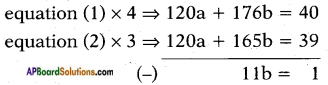⇒ b = $$\frac{1}{11}$$
Substituting b = $$\frac{1}{11}$$ in equation (1) we get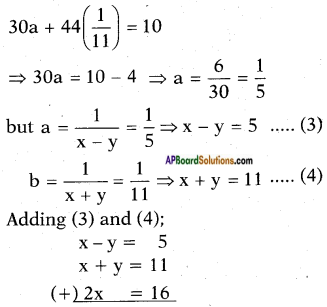⇒ x = 8
Substituting x = 8 in x – y = 5 we get
8 – y = 5
⇒ y = 8 – 5 = 3
∴ The solution (x, y) = (8, 3)
Speed of the boat in still water = 8 kmph
Speed of the stream = 3 kmph.ii) Rahim travels 600 km to his home partly by train and partly by car. He takes 8 hours if he travels 120 km by train and rest by car. He takes 20 minutes more if he travels 200 km by train and rest by car. Find the speed of the train and the car.
Let the speed of the train be x kmph
and the speed of the car = y kmph
By problem,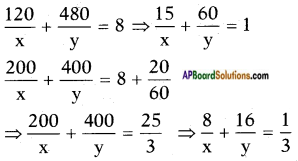Take $$\frac{1}{x}$$ = a and $$\frac{1}{y}$$ = b, then
the given equations reduce to
15a + 60b = 1 ……… (1)
8a + 16b = $$\frac{1}{3}$$ ⇒ 24a + 48b = 1 ……… (2)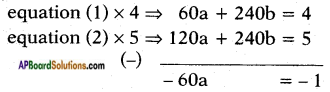⇒ a = $$\frac{-1}{-60}$$ = $$\frac{1}{60}$$
Substituting a = $$\frac{1}{60}$$ in equation (1) we get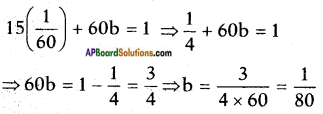but a = $$\frac{1}{x}$$ = $$\frac{1}{60}$$ ⇒ x = 60 kmph
b = $$\frac{1}{y}$$ = $$\frac{1}{80}$$ ⇒ y = 80 kmph
Speed of the train = 60 kmph and
speed of the car = 80 kmph

iii) 2 women and 5 men can together finish an embroidery work in 4 days while 3 women and 6 men can finish it in 3 days. Find the time taken by 1 woman alone and 1 man alone to finish the work.
Let the time taken by 1 woman to complete the work = x days
and time taken by 1 man to complete the work = y days
∴ Work done by 1 woman in 1 day = $$\frac{1}{x}$$
Work done by 1 man in 1 day = $$\frac{1}{y}$$
By problem,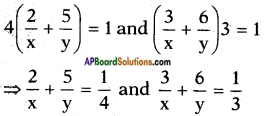Take $$\frac{1}{x}$$ = a and $$\frac{1}{y}$$ = b,
then the above equations reduce to
2a + 5b = $$\frac{1}{4}$$ and 3a + 6b = $$\frac{1}{3}$$
⇒ 8a + 20b = 1 …….. (1) and
9a + 18b = 1 ……… (2)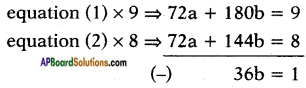⇒ b = $$\frac{1}{36}$$
Substituting b = $$\frac{1}{36}$$ in equation (1) we get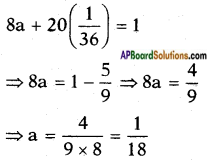but a = $$\frac{1}{x}$$ = $$\frac{1}{18}$$ ⇒ x = 18 and
b = $$\frac{1}{y}$$ = $$\frac{1}{36}$$ ⇒ y = 36
∴ Time taken by 1 woman = 18 days
1 man = 36 days